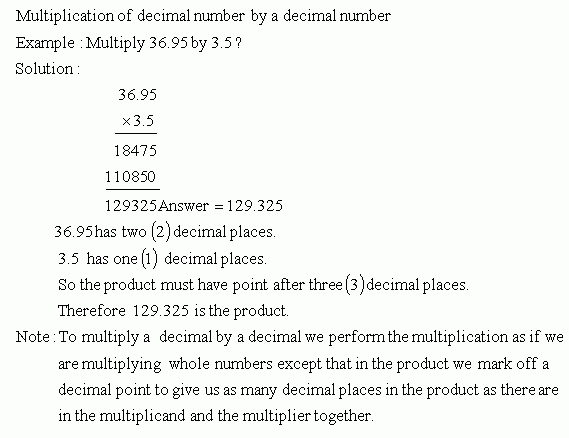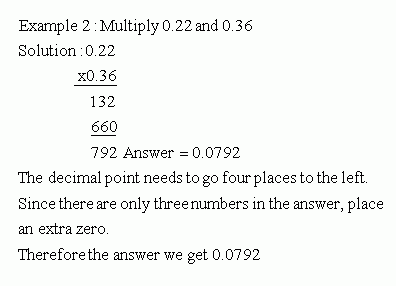Name: ___________________Date:___________________

 Email us to get an instant 20% discount on highly effective K-12 Math & English kwizNET Programs!

### Grade 6 - Mathematics5.7 Multiplication In Decimals - (Multiply by Decimal Number)Directions: Solve the following problems. Also write at least 10 examples of your own.Name: ___________________Date:___________________

### Grade 6 - Mathematics5.7 Multiplication In Decimals - (Multiply by Decimal Number)

 Q 1: 0.7682 x 2.7 =Answer: Q 2: 790.7 x 5.61 =Answer: Q 3: 256.28 x 1.2 =Answer: Q 4: 0.789 x 0.2 =Answer: Q 5: 780.29 x 4.5 =Answer: Q 6: 76.278 x 0.76 =Answer: Q 7: 789.67 x 3.4 =Answer: Q 8: 2670.78 x 6.7 =Answer: Question 9: This question is available to subscribers only! Question 10: This question is available to subscribers only!

#### Subscription to kwizNET Learning System costs less than \$1 per month & offers the following benefits:

• Unrestricted access to grade appropriate lessons, quizzes, & printable worksheets
• Instant scoring of online quizzes
• Progress tracking and award certificates to keep your student motivated
• Unlimited practice with auto-generated 'WIZ MATH' quizzes